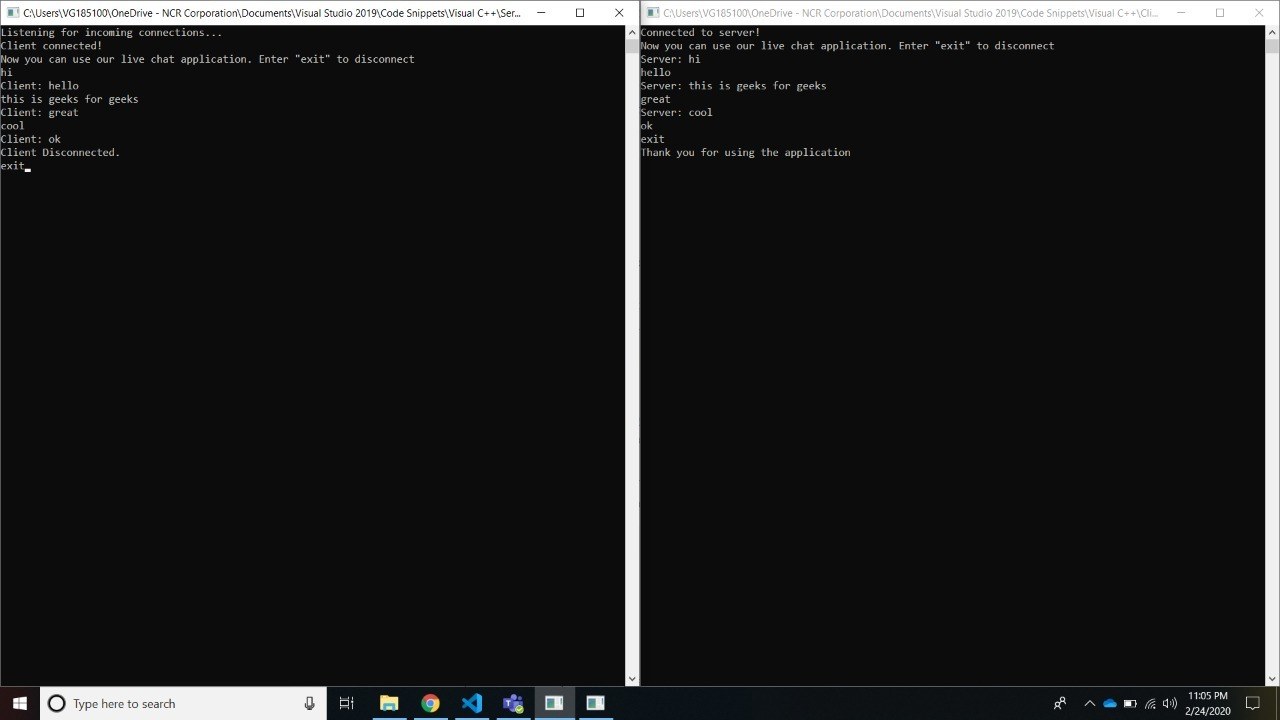# Two way communication between Client and Server using Win32 Threads

• Difficulty Level : Hard
• Last Updated : 26 Jul, 2021

Prerequisite: Socket Programming in C/C++
It is possible to send data from the server and receive a response from the client. Similarly, the client can also send and receive data to-and-from. Here we will discuss the approach using Win32 Threads in C/C++.

Approach:

1. Use CreateThread function which creates a new thread for a process.
2. The CreateThread method must specify the starting address of the code that the new thread is to execute. Following is the prototype of CreateThread function:
```HANDLE CreateThread(
DWORD dwStackSize,
LPVOID lpParameter, DWORD dwCreationFlags,
);
```
3. Then using WaitForSingleObject function that returns message in form of object received from client, to receive data from client. Following is the prototype of WaitForSingleObject function:
```DWORD WaitForSingleObject(
HANDLE hHandle,
DWORD dwMilliseconds
);
```

Creating the Server program
In the Server Program, we will be using two threads, one for Sending data to the client and another for Receiving data from the client. The process of communication stops when both Client and Server type “exit“.

Below is the implementation of the Server Program:

## Server

 `// C++ program to create Server`` ` `#include ``#include ``#include ``using` `namespace` `std;`` ` `// Function that receive data``// from client``DWORD` `WINAPI serverReceive(``LPVOID` `lpParam)``{``    ``// Created buffer[] to``    ``// receive message``    ``char` `buffer = { 0 };`` ` `    ``// Created client socket``    ``SOCKET client = *(SOCKET*)lpParam;`` ` `    ``// Server executes continuously``    ``while` `(``true``) {`` ` `        ``// If received buffer gives``        ``// error then return -1``        ``if` `(recv(client, buffer, ``sizeof``(buffer), 0)``            ``== SOCKET_ERROR) {``            ``cout << ``"recv function failed with error "``                 ``<< WSAGetLastError() << endl;``            ``return` `-1;``        ``}`` ` `        ``// If Client exits``        ``if` `(``strcmp``(buffer, ``"exit"``) == 0) {``            ``cout << ``"Client Disconnected."``                 ``<< endl;``            ``break``;``        ``}`` ` `        ``// Print the message``        ``// given by client that``        ``// was stored in buffer``        ``cout << ``"Client: "` `<< buffer << endl;`` ` `        ``// Clear buffer message``        ``memset``(buffer, 0,``               ``sizeof``(buffer));``    ``}``    ``return` `1;``}`` ` `// Function that sends data to client``DWORD` `WINAPI serverSend(``LPVOID` `lpParam)``{``    ``// Created buffer[] to``    ``// receive message``    ``char` `buffer = { 0 };`` ` `    ``// Created client socket``    ``SOCKET client = *(SOCKET*)lpParam;`` ` `    ``// Server executes continuously``    ``while` `(``true``) {`` ` `        ``// Input message server``        ``// wants to send to client``        ``gets``(buffer);`` ` `        ``// If sending failed``        ``// return -1``        ``if` `(send(client,``                 ``buffer,``                 ``sizeof``(buffer), 0)``            ``== SOCKET_ERROR) {``            ``cout << ``"send failed with error "``                 ``<< WSAGetLastError() << endl;``            ``return` `-1;``        ``}`` ` `        ``// If server exit``        ``if` `(``strcmp``(buffer, ``"exit"``) == 0) {``            ``cout << ``"Thank you for using the application"``                 ``<< endl;``            ``break``;``        ``}``    ``}``    ``return` `1;``}`` ` `// Driver Code``int` `main()``{``    ``// Data``    ``WSADATA WSAData;`` ` `    ``// Created socket server``    ``// and client``    ``SOCKET server, client;`` ` `    ``// Socket address for server``    ``// and client``    ``SOCKADDR_IN serverAddr, clientAddr;`` ` `    ``WSAStartup(MAKEWORD(2, 0), &WSAData);`` ` `    ``// Making server``    ``server = socket(AF_INET,``                    ``SOCK_STREAM, 0);`` ` `    ``// If invalid socket created,``    ``// return -1``    ``if` `(server == INVALID_SOCKET) {``        ``cout << ``"Socket creation failed with error:"``             ``<< WSAGetLastError() << endl;``        ``return` `-1;``    ``}``    ``serverAddr.sin_addr.s_addr = INADDR_ANY;``    ``serverAddr.sin_family = AF_INET;``    ``serverAddr.sin_port = htons(5555);`` ` `    ``// If socket error occurred,``    ``// return -1``    ``if` `(bind(server,``             ``(SOCKADDR*)&serverAddr,``             ``sizeof``(serverAddr))``        ``== SOCKET_ERROR) {``        ``cout << ``"Bind function failed with error: "``             ``<< WSAGetLastError() << endl;``        ``return` `-1;``    ``}`` ` `    ``// Get the request from``    ``// server``    ``if` `(listen(server, 0)``        ``== SOCKET_ERROR) {``        ``cout << ``"Listen function failed with error:"``             ``<< WSAGetLastError() << endl;``        ``return` `-1;``    ``}`` ` `    ``cout << "Listening ``for` `            ``incoming connections...." << endl;`` ` `            ``// Create buffer[]``            ``char` `buffer;`` ` `    ``// Initialize client address``    ``int` `clientAddrSize = ``sizeof``(clientAddr);`` ` `    ``// If connection established``    ``if` `((client = accept(server,``                         ``(SOCKADDR*)&clientAddr,``                         ``&clientAddrSize))``        ``!= INVALID_SOCKET) {``        ``cout << ``"Client connected!"` `<< endl;``        ``cout << ``"Now you can use our live chat application."``             ``<< ``"Enter \"exit\" to disconnect"` `<< endl;`` ` `        ``// Create variable of``        ``// type DWORD``        ``DWORD` `tid;`` ` `        ``// Create Thread t1``        ``HANDLE` `t1 = CreateThread(NULL,``                                 ``0,``                                 ``serverReceive,``                                 ``&client,``                                 ``0,``                                 ``&tid);`` ` `        ``// If created thread``        ``// is not created``        ``if` `(t1 == NULL) {``            ``cout << ``"Thread Creation Error: "``                 ``<< WSAGetLastError() << endl;``        ``}`` ` `        ``// Create Thread t2``        ``HANDLE` `t2 = CreateThread(NULL,``                                 ``0,``                                 ``serverSend,``                                 ``&client,``                                 ``0,``                                 ``&tid);`` ` `        ``// If created thread``        ``// is not created``        ``if` `(t2 == NULL) {``            ``cout << ``"Thread Creation Error: "``                 ``<< WSAGetLastError() << endl;``        ``}`` ` `        ``// Received Objects``        ``// from client``        ``WaitForSingleObject(t1,``                            ``INFINITE);``        ``WaitForSingleObject(t2,``                            ``INFINITE);`` ` `        ``// Close the socket``        ``closesocket(client);`` ` `        ``// If socket closing``        ``// failed.``        ``if` `(closesocket(server)``            ``== SOCKET_ERROR) {``            ``cout << ``"Close socket failed with error: "``                 ``<< WSAGetLastError() << endl;``            ``return` `-1;``        ``}``        ``WSACleanup();``    ``}``}`

Run the ServerApplication.cpp file using the command:
```g++ ServerApplication.cpp -lws2_32
```

Creating the Client Program
In the Client Program, we will be using two threads one for Sending data to the server and another for Receiving data from the server. The process of communication stops when both Server and Client type “exit“.

## Client

 `// C++ program to create client`` ` `#include ``#include ``#include ``using` `namespace` `std;`` ` `// Function that receive data from server``DWORD` `WINAPI clientReceive(``LPVOID` `lpParam)``{``    ``// Created buffer[] to``    ``// receive message``    ``char` `buffer = { 0 };`` ` `    ``// Created server socket``    ``SOCKET server = *(SOCKET*)lpParam;`` ` `    ``// Client executes continuously``    ``while` `(``true``) {`` ` `        ``// If received buffer gives``        ``// error then return -1``        ``if` `(recv(server, buffer,``                 ``sizeof``(buffer), 0)``            ``== SOCKET_ERROR) {``            ``cout << ``"recv function failed with error: "``                 ``<< WSAGetLastError()``                 ``<< endl;``            ``return` `-1;``        ``}`` ` `        ``// If Server exits``        ``if` `(``strcmp``(buffer, ``"exit"``) == 0) {``            ``cout << ``"Server disconnected."``                 ``<< endl;``            ``return` `1;``        ``}`` ` `        ``// Print the message``        ``// given by server that``        ``// was stored in buffer``        ``cout << ``"Server: "` `<< buffer << endl;`` ` `        ``// Clear buffer message``        ``memset``(buffer, 0, ``sizeof``(buffer));``    ``}``    ``return` `1;``}`` ` `// Function that sends data to server``DWORD` `WINAPI clientSend(``LPVOID` `lpParam)``{``    ``// Created buffer[] to``    ``// receive message``    ``char` `buffer = { 0 };`` ` `    ``// Created server socket``    ``SOCKET server = *(SOCKET*)lpParam;`` ` `    ``// Client executes continuously``    ``while` `(``true``) {`` ` `        ``// Input message client``        ``// wants to send to server``        ``gets``(buffer);`` ` `        ``// If sending failed``        ``// return -1``        ``if` `(send(server,``                 ``buffer,``                 ``sizeof``(buffer), 0)``            ``== SOCKET_ERROR) {``            ``cout << ``"send failed with error: "``                 ``<< WSAGetLastError() << endl;``            ``return` `-1;``        ``}`` ` `        ``// If client exit``        ``if` `(``strcmp``(buffer, ``"exit"``)``            ``== 0) {``            ``cout << ``"Thank you for using the application"``                 ``<< endl;``            ``break``;``        ``}``    ``}``    ``return` `1;``}`` ` `// Driver Code``int` `main()``{``    ``// Input data``    ``WSADATA WSAData;`` ` `    ``// Created socket server``    ``SOCKET server;``    ``SOCKADDR_IN addr;`` ` `    ``WSAStartup(MAKEWORD(2, 0), &WSAData);`` ` `    ``// If invalid socket created,``    ``// return -1``    ``if` `((server = socket(AF_INET,``                         ``SOCK_STREAM, 0))``        ``== INVALID_SOCKET) {``        ``cout << ``"Socket creation failed with error: "``             ``<< WSAGetLastError() << endl;``        ``return` `-1;``    ``}`` ` `    ``addr.sin_addr.s_addr = inet_addr(``"127.0.0.1"``);``    ``addr.sin_family = AF_INET;``    ``addr.sin_port = htons(5555);`` ` `    ``// If connection failed``    ``if` `(connect(server,``                ``(SOCKADDR*)&addr,``                ``sizeof``(addr))``        ``== SOCKET_ERROR) {``        ``cout << ``"Server connection failed with error: "``             ``<< WSAGetLastError() << endl;``        ``return` `-1;``    ``}`` ` `    ``// If connection established``    ``cout << ``"Connected to server!"``         ``<< endl;``    ``cout << ``"Now you can use our live chat application."``         ``<< ``" Enter \"exit\" to disconnect"``         ``<< endl;``    ``DWORD` `tid;`` ` `    ``// Create Thread t1``    ``HANDLE` `t1 = CreateThread(NULL,``                             ``0,``                             ``clientReceive,``                             ``&server,``                             ``0, &tid);`` ` `    ``// If created thread``    ``// is not created``    ``if` `(t1 == NULL)``        ``cout << ``"Thread creation error: "``             ``<< GetLastError();`` ` `    ``// Create Thread t2``    ``HANDLE` `t2 = CreateThread(NULL,``                             ``0,``                             ``clientSend,``                             ``&server,``                             ``0, &tid);`` ` `    ``// If created thread``    ``// is not created``    ``if` `(t2 == NULL)``        ``cout << ``"Thread creation error: "``             ``<< GetLastError();`` ` `    ``// Received Objects``    ``// from client``    ``WaitForSingleObject(t1, INFINITE);``    ``WaitForSingleObject(t2, INFINITE);`` ` `    ``// Socket closed``    ``closesocket(server);``    ``WSACleanup();``}`

Run the ClientApplication.cpp file using the command:

```g++ ClientApplication.cpp -lws2_32
```

Output After communication between Server and ClientThe left side command prompt is the ServerApplication and the right side command prompt is the ClientApplication.

My Personal Notes arrow_drop_up